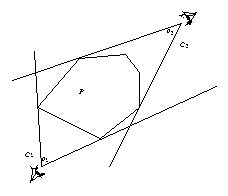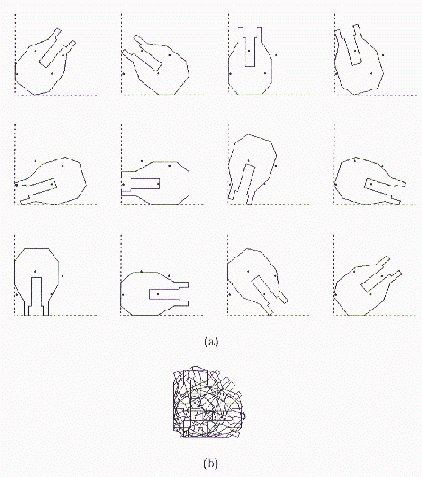# Robotics Laboratory

## Sensing Based on Geometric Constraints

### (a) Polygon Inscription

In my Ph.D. research I investigated geometric and mechanical sensing strategies for objects of known shapes. Examples include industrial parts and everyday desktop items. In carrying out this work, I endeavored to understand the evolution and encoding of information in robot tasks subject to their geometry and mechanics, and based on such understanding, to determine their (minimum) information requirements for physical sensors. Sample questions in my mind included: What can be sensed in a task? How much information is enough? How to interpret sensor data? How to implement physical sensors?

To seek the answers, I looked into physical sensing approaches such as probing and cone inscription as well as manipulation operations such as pushing and rolling. My research drew upon techniques from differential geometry, computational geometry, algorithm design, computational complexity theory, nonlinear control, numerical methods, mechanics, as well as sensor design.

One type of sensing employs simple sensors to obtain geometric constraints that can either immobilize a part or distinguish one possible pose (position and orientation) from others in a finite set, and then solves a constraint satisfaction problem for the pose using an efficient algorithm. The shapes are assumed to be known in advance.Under the supervision of Mike Erdmann, the first problem I looked at involves detecting the pose of a convex polygon in the plane by taking views of the polygon from multiple exterior sites (as illustrated in the figure on the right). Each view results in a cone formed by the outermost occluding rays starting from the viewing site. The cone in turn imposes a constraint on the possible poses of the polygon, which must be contained in the cone and make contact with both its sides. Such a containment is called inscription. An algorithm with running time O(nm), where n is the number of polygon vertices and m the number of cones, was presented to solve for all possible poses of the polygon. In practice the algorithm runs in time O(n + m log n). I also proved that the number of possible poses cannot exceed 6n, given m>=2 supporting cones with distinct vertices.

Simulations demonstrated that two supporting cones are sufficient for determining the real polygon pose in most cases. The results imply that sensing in practice can be carried out by obtaining viewing angles of a planar part at multiple exterior sites in the plane (or solid angles subtended by a 3-dimensional part at different locations in the space). An implementation may use a rotary sensor or a linear CCD array combined with a diverging lens to obtain the necessary constraints.

More details can be found in the paper:

• Yan-Bin Jia and Michael Erdmann. Sensing polygon poses by inscription. In Proceedings of the 1994 IEEE International Conference on Robotics and Automation, pp. 1642-1649, San Diego, CA, May 1994.

The above work addresses at least the following issues of geometric sensing:

• What can be sensed? (Geometric constraints)
• How much data is necessary? (At least locally constraining the pose)
• How to recover the pose? (Constraint satisfaction algorithms)
• How efficient? (Asymptotic running time)
• Sensing ambiguities? (Worst case analysis)

### (b) Point SamplingOn many occasions a parts feeder will have reduced the number of possible poses of a part to a small finite set. For illustration, consider a polygonal part resting on a horizontal assembly table shown on the right. The table is bounded by vertical fences at its bottom left corner. Pushing the part toward that corner will eventually cause the part to settle in one of the 12 stable poses. To distinguish between these 12 poses, the robot has marked 4 points on the table beforehand, so it can infer the pose from which marks are covered and which are not.

Intuitively, each sensing point can be regarded as a binary bit that has two values ‘contained’ and ‘not contained’. So the robot senses a shape by reading out the binary representation of the shape, that is, by checking which points are contained in the shape and which are not. Thus the formalized sensing problem: Given n polygons with a total of m edges in the plane, locate the fewest points such that each polygon contains a distinct subset of points in its interior. We showed that this problem is equivalent to an NP-complete set-theoretic problem introduced as Discriminating Set, and present an O(n^2 m^2) approximation algorithm to solve it with a ratio of 2 lnn. Furthermore, we proved that one can use an algorithm for Discriminating Set with ratio c log n to construct an algorithm for Set Covering with ratio c log n + O(log log n). Thus the ratio 2 ln n is asymptotically optimal unless the class NP is a subset of the class DTIME), a consequence of known results on approximating Set Covering.

In practice, light detectors may be placed underneath the precomputed sampling locations on the surface of an assembly table or in a tray. The actual embedding can be avoided if the surface is transparent. In this case light detectors can be easily reconfigured given different sets of parts and poses, which ensures the modularity of sensing. Another implementation strategy is to mechanically probe the sampling points using a robot. Robustness of sampling points is more important since even a slight probe may disturb the object’s pose.

We refer the reader to the following paper for more details:

• Yan-Bin Jia and Michael Erdmann. The complexity of sensing by point sampling. In Algorithmic Foundations of Robotics, Ken Goldberg et al. (eds.), pp. 283-300, A. K. Peters, Boston, 1995. Also presented at the First International Workshop on the Algorithmic Foundations of Robotics, San Francisco, CA, February 1998.

We have viewed the cost of geometric sensing as the composition of the sensor cost and the time cost, the latter of which in turn consists of the costs of physical operations and computations. The size of geometric constraints is proportional to the sensor data and thus, depending on the implementation, is proportional to the number of sensors or the number of physical operations. Our work on point sampling is an example of how to tackle the following issues surrounding the minimization of geometric constraints:

• Hardness. (Polynomial time solvable?)
• If hard, how to efficiently compute suboptimal constraints? (Approximation)

The results on inscription and point sampling were later refined and combined into the journal paper: